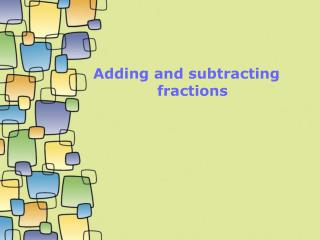DownloadDownload PresentationDownload Presentation- - - - - - - - - - - - - - - - - - - - - - - - - - - E N D - - - - - - - - - - - - - - - - - - - - - - - - - - -
##### Presentation Transcript

2. Fractions with the same denominator Check the answers to these questions on your calculator: a. b. c. d.

3. Fractions with the same denominator Check the answers to these questions on your calculator: a. b. c. d. Did you see that you simply add the numerators when the denominators are the same?

4. Write down the answers to these questions: a. b. c. d. Check on your calculator if you are not sure of your answers.

5. Did you get them correct? a. b. c. d.

6. Fractions with different denominators Check the answers to these questions on your calculator: a. b. c. d.

7. Fractions with different denominators Check the answers to these questions on your calculator: a. b. c. d. Did you see that when the denominators are different you DO NOT simply add the numerators?

8. Fractions with different denominators a. b. c. d. Did you get them correct?

9. To be able to add these two fractions the denominators need to be the same!...... Can you make up a rule which works for this sort of question??

10. Does your rule work for these questions? Check on your calculator a. b. c. d.

11. Did you get them correct a. b. c. d.

12. Did your rule work??? a. b. c. d.

13. How does this work? To add fractions with different denominators we need to make use of equivalent fractions to write them with the same denominator.

14. How does this work? To add fractions with different denominators we need to make use of equivalent fractions to write them with the same denominator. To change to have a denominator of 15, we need to multiply by the fraction . Why?

15. How does this work? To add fractions with different denominators we need to make use of equivalent fractions to write them with the same denominator. To change to have a denominator of 15, we need to multiply by the fraction . Then

16. How does this work? To add fractions with different denominators we need to make use of equivalent fractions to write them with the same denominator. To change to have a denominator of 15, we need to multiply by the fraction . Then So to calculate we need to express each fraction with denominator 15 first.

17. Check these steps carefully

18. Backdrops: - These are full sized backdrops, just scale them up! - Can be Copy-Pasted out of Templates for use anywhere! www.animationfactory.com